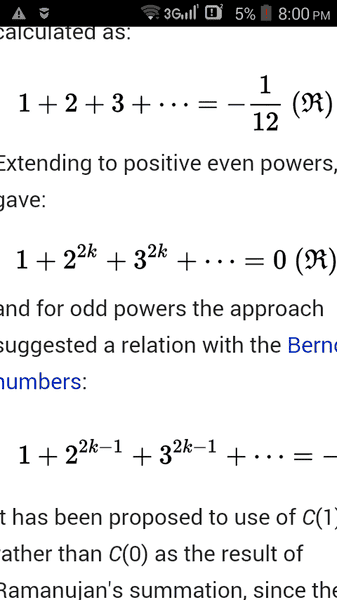# 1+4+9+16+...=0 proof

• I
Hello, I started to learn divergent series/sums, to practice I calculated some basic ones, you know: 1+2+3+4+5+6...= -1/12, but I really had problems when i tried to demonstrate that 1+4+9+16+...= 0(the sum of squares of natural numbers), I've tried to add, subtract etc, but I couldn't prove it, anyone here could help?

mfb
Mentor
I've tried to add, subtract etc, but I couldn't prove it, anyone here could help?
I guess you are referring to things like this? Forget it. Those calculations are mathematically nonsense, and you can get any result you like if you use those wrong operations. They are not the way those pseudo-limits are defined.

As an example, consider
1+1+1+1+.... = X
Let's add 1 to both sides:
1+1+1+1+... = 1+X
But the left sides of both equations are identical, therefore, X=1+X.
Subtract X:
0=1.

I guess you are referring to things like this? Forget it. Those calculations are mathematically nonsense, and you can get any result you like if you use those wrong operations. They are not the way those pseudo-limits are defined.
S= 1-2+3-4+5... And adding S+S, it'll eventually give us 2*S=1-1+1-1+...
That's how we can assign values to these series => S= 1/4 (1-1+1-1+1...= 1/2 )

PeroK
Homework Helper
Gold Member
2021 Award
Hello, I started to learn divergent series/sums, to practice I calculated some basic ones, you know: 1+2+3+4+5+6...= -1/12, but I really had problems when i tried to demonstrate that 1+4+9+16+...= 0(the sum of squares of natural numbers), I've tried to add, subtract etc, but I couldn't prove it, anyone here could help?

What about ##1 + 4 + 9 + 16 \dots = 1 +(-1 + 1) + 4 + (-5 + 5) + 9 + (-14 + 14) + 16 + (-30 + 30) \dots = 0 + 5 - 5 + 14 - 14 + 30 - 30 + \dots = 0 + 0 + 0 + 0 \dots = 0##

Although:

##1 + 2 + 3 + 4 + 5 \dots = 1 + (-1 + 1) + 2 + (-3 + 3) + 4 + (-7 + 7) + 5 + (-12 + 12) \dots) = 0##

Looks like they all sum to 0.

mfb
Mentor
That's how we can assign values to these series => S= 1/4 (1-1+1-1+1...= 1/2 )
You can, but those values are meaningless. See the example I put in my post, you can easily make contradictions like that.

It is not what mathematicians do to assign values to those series!

As an example, consider
1+1+1+1+.... = X
Let's add 1 to both sides:
1+1+1+1+... = 1+X
But the left sides of both equations are identical, therefore, X=1+X.
Subtract X:
0=1.
I wrote that I add sums, in general, mathematicians do that, but in this example you added 1 to an infinite series that's wrong, infinity + 1 = infinity.

As written in that wikipedia article mathematician 'assign' the values to series, maybe equal is much said, but how to assign 0 to the 1+4+9+... would be a better formulation of the question.

This method of assigning is used in many papers(including string theory).
A video from Numberphile would explain visually what I mean:
but I would like to know how to do this for 1+4+9+... series.

ShayanJ
Gold Member
See this series of videos!
I don't remember in which, but he does explain assigning values to divergent series.

mfb
Mentor
I wrote that I add sums, in general, mathematicians do that
Not in the way you wrote here, no.
but in this example you added 1 to an infinite series that's wrong
That is my point, the operations you do with the series are wrong.
As written in that wikipedia article mathematician 'assign' the values to series, maybe equal is much said, but how to assign 0 to the 1+4+9+... would be a better formulation of the question.
There are various methods, in this particular case it is Ramanujan summation. More options (which can lead to different answers for the same series) are listed here.

•DrewD
Not in the way you wrote here, no
How would be a correct way to write it?
There are various methods, in this particular case it is Ramanujan summation.I would like to know the steps he applied to be able to assign 0 to the middle series.
[PLAIN]http://QUOTE="mfb, [Broken] post: 5498284, member: 405866"]Not in the way you wrote here, no[/QUOTE] [/PLAIN]

Last edited by a moderator:
mfb
Mentor
I would like to know the steps he applied to be able to assign 0 to the middle series.
See the section above, "Summation".

Mark44
Mentor
As mfb said,
Those calculations are mathematically nonsense
This misuse of divergent series has been discussed numerous times here at PF.